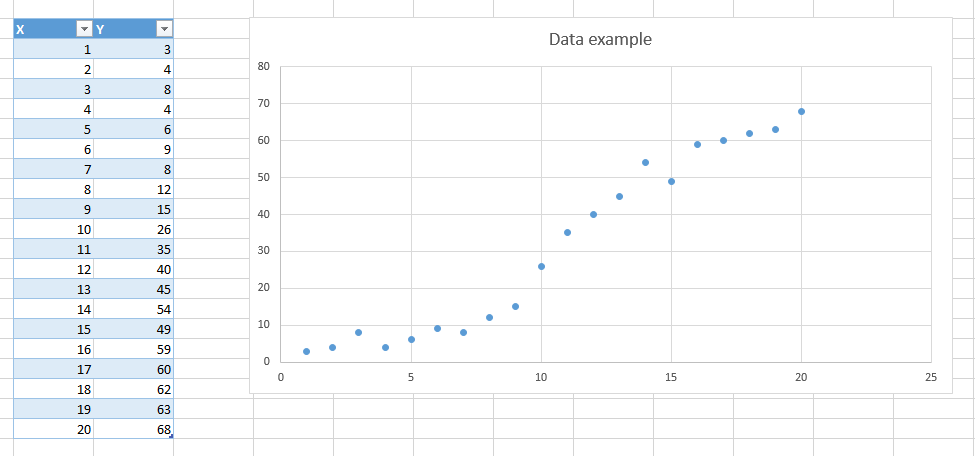## Support Vector Regression with R

In this article I will show how to use R to perform a Support Vector Regression.
We will first do a simple linear regression, then move to the Support Vector Regression so that you can see how the two behave with the same data.

## A simple data set

To begin with we will use this simple data set:I just put some data in excel. I prefer that over using an existing well-known data-set because the purpose of the article is not about the data, but more about the models we will use.

As you can see there seems to be some kind of relation between our two variables X and Y, and it look like we could fit a line which would pass near each point.

Let's do that in R !